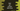# How to get the current date-time in Dart## How to get the current date-time data in dart:

Dart provides DateTime class to deal with date and time. We can use this class to print the current date-time info and different values of the current date and time.

### DateTime.now() :

now() method is a method in the DateTime class that is used to create a DateTime object in the current date and time in the local time zone. Let’s take a look at the below program:

``````void main() {
print(new DateTime.now());
}``````

It will print the below output:

``2020-12-18 19:20:28.130251``

### Other properties:

We can also use these properties: .day: This is an integer value that defines the day of the month. It lies in 1 to 31 .hour: This is an integer value in 24 hour clock. It is in 0 to 23 .minute: This is an integer value in range 0 to 59 .month: The month value in integer, range is in 1 to 12 .second: The second value in integer, range is in 0 to 59 .weekday: The day of the week in integer .year: Year in integer

Let’s take a look at the below example:

``````void main() {
var currentDate = new DateTime.now();

print(currentDate);
print("day :"+currentDate.day.toString());
print("hour :"+currentDate.hour.toString());
print("minute :"+currentDate.minute.toString());
print("month :"+currentDate.month.toString());
print("second :"+currentDate.second.toString());
print("weekday :"+currentDate.weekday.toString());
print("year :"+currentDate.year.toString());
}``````

It will print:

``````2021-01-26 19:35:29.694845
day :26
hour :19
minute :35
month :1
second :29
weekday :2
year :2021``````